Published: 15 February 2017

Lei-an Zhang1
Li-ming Tao2
Xiu-ting Wei3
Xue-mei Huang4
1, 2, 3, 4College of Mechanical Engineering, Shandong University of Technology, Shandong, China
Corresponding Author:
Lei-an Zhang
Views 182

## 1. Introduction

Fig. 1Distribution curve of phase angle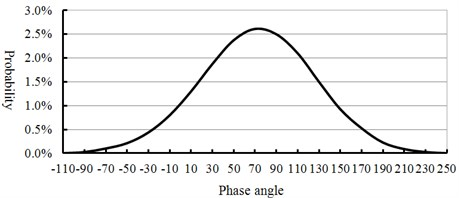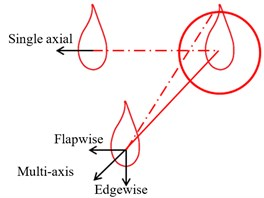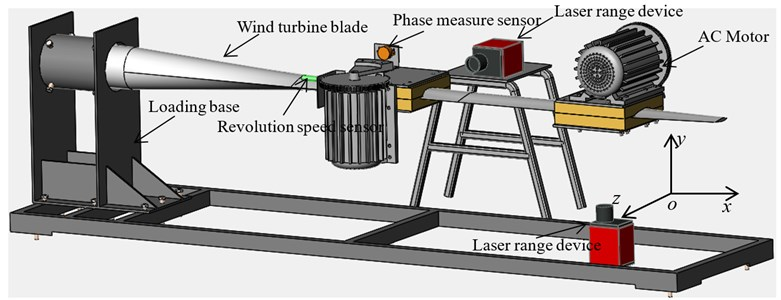### 2.2. Measurement of speed difference, phase difference and amplitude

High counting sensor which adopting advanced detection technology could identify every teeth of gear and convert it into a square wave output. Output shaft of each loading source installed with a measuring gear and two sensors measuring the revolution speed and phase angle, as shown in Fig. 4. The speed measuring gear had 180 teeth and each of which represented two angles. There was a zero mark on the speed measuring gear. During the measuring, each gear speed sensor produced a pulse when a tooth of gear was detected, so the driving speeds and phases of the unbalanced shafts could be obtained. A proximity switch is installed below every unbalanced shaft to realize the reset of phase.

Fig. 4Speed difference and phase difference detection scheme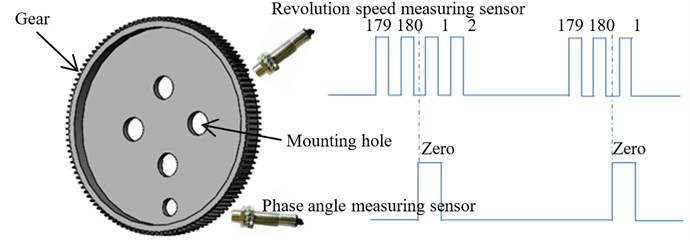The centrifugal force generated by each eccentric mass was defined as the center of gravity line (denoted by ${M}_{1}$ and ${M}_{2}$). The two center line of gravity could be maintained at a constant angle, which indicated that the two loading source vibrated synchronization. Take the two loading sources for example, the revolution speed difference $\mathrm{\Delta }v\left(t\right)$ (rad/s) and phase angle difference $\mathrm{\Delta }\phi \left(t\right)$ (rad) could be expressed as:

1
$\mathrm{\Delta }v\left(t\right)={v}_{1}\left(t\right)-{v}_{2}\left(t\right)=\frac{d{M}_{1}\left(t\right)-d{M}_{2}\left(t\right)}{dt},$
2
$\mathrm{\Delta }M\left(t\right)=\mathrm{\Delta }M\left(0\right)+\int \mathrm{\Delta }v\left(t\right)tdtm$
3
$\mathrm{\Delta }\phi \left(t\right)=\left\{\begin{array}{ll}\mathrm{\Delta }M\left(t\right)-2\pi ,& \mathrm{\Delta }M\left(t\right)\ge 2\pi ,\\ \mathrm{\Delta }M\left(t\right)+2\pi ,& \mathrm{\Delta }M\left(t\right)\le -2\pi ,\\ \mathrm{\Delta }M\left(t\right),& -2\pi <\mathrm{\Delta }M\left(t\right)<2\pi ,\end{array}\right\$

where: ${v}_{1}\left(t\right)$, ${v}_{2}\left(t\right)$ – revolution speed of eccentric mass; ${M}_{1}\left(t\right)$, ${M}_{2}\left(t\right)$ – the position of eccentric mass; $\mathrm{\Delta }\phi \left(t\right)$ – phase angle difference; $\mathrm{\Delta }M\left(0\right)$ – initial position.

The differential detection of revolution speed and phase angle could be realized according to Eqs. (1)-(3).

Non-contact laser measurement was used to avoid damage for the measuring equipment because of the blade vibration. Measurement equipment was laser range device. The laser range mode was set a one-way transmission mode and communication format was set to: 19200.EVEN.8.1. Data transmission cycle was $T=$30 ms. The blade amplitude was represented by three bytes. The commutation protocol was shown in Table 1.

Table 1Laser ranging protocol

 7bit 6bit 5bit 4bit 3bit 2bit 1bit 0bit Data (L) 0 0 7bit 6bit 5bit 4bit 3bit 2bit 1bit 0bit Data (M) 0 1 7bit 6bit 5bit 4bit 3bit 2bit 1bit 0bit × × Data (H) 1 0

According to the communication protocol of Table 1, the formula of blade amplitude was calculated as follows: Amplitude = (L$\gg$2)+(M$\gg$2)*64+((H$\gg$2)&0×0F)*4096;

## 3. Electromechanical coupling dynamic model

### 3.1. Mathematical model

According to the multi-axis loading scheme of wind turbine as shown in Fig. 3, some assumptions were made as the following:

(2) When the blade vibrates, damping force and elastic force were proportional to the velocity and displacement of the blade respectively.

(3) $xoy$ plane was in the blade oriented direction, and $xoz$ plane was in the blade tangential direction.

Three coordinate systems and five degree freedoms were defined: translatory motion along the $x$ axis, $y$ axis, $z$ axis and rotation around the $x$ axis, $z$ axis respectively. The proposed Dynamic model as shown in Fig. 5.

As shown in Fig. 5, $oxyz$ was the absolute coordinate system, ${o}^{\text{'}}{x}^{\text{'}}{y}^{\text{'}}{z}^{\text{'}}$ and ${o}^{\text{'}\text{'}}{x}^{\text{'}\text{'}}{y}^{\text{'}\text{'}}{z}^{\text{'}\text{'}}$ were the moving coordinate systems, ${o}^{\text{'}}\left({o}^{\text{'}\text{'}}\right)$ was motion synthesis centroid of the system; ${o}^{\text{'}\text{'}\text{'}}$ was the centroid of the whole vibration system (including the blade and the two loading sources). In addition, $M$ was the equivalent weight of the blade; ${o}_{1}$ and ${o}_{2}$ were the rotary center of two eccentric mass respectively. And, ${l}_{1}$and ${l}_{1}^{\text{'}}$ were the projection of $o{o}_{1}$ in the plane $xoy$ and $yoz$ respectively; ${l}_{2}$ and ${l}_{2}^{\text{'}}$ were the projection of $o{o}_{2}$ in the plane $xoy$ and $yoz$ respectively. The angle between ${l}_{1}$, ${l}_{2}$ were ${\beta }_{1}^{}$ and ${\beta }_{2}^{}$and the positive direction of $x$-axis respectively. ${\beta }_{1}^{\text{'}}$ was the angle between ${l}_{1}^{\text{'}}$ and the negative direction of $z$-axis and ${\beta }_{2}^{\text{'}}$ was the angle between ${l}_{2}^{\text{'}}$ and the positive direction of $z$-axis; ${m}_{i}$($i=$1, 2) was the equivalent weight of the eccentric mass; ${r}_{i}$($i=$1, 2) was the equivalent eccentricity of the eccentric mass; ${\theta }_{1}$ and ${\theta }_{2}$were the rotation angle of the eccentric mass respectively, and ${\omega }_{i}=d{\theta }_{i}/dt$ ($i=$1, 2); $\phi$ was the rise angle of connecting rod ${m}_{2}$.

The kinetic energy $T$ of the proposed system was expressed as:

4
$T=\frac{1}{2}M\left[\left(\stackrel{˙}{x}+{\stackrel{˙}{\phi }}_{1}{l}_{0}\mathrm{s}\mathrm{i}\mathrm{n}{\beta }_{0}{\right)}^{2}+\left(\stackrel{˙}{y}-{\stackrel{˙}{\phi }}_{1}{l}_{0}\mathrm{c}\mathrm{o}\mathrm{s}{\beta }_{0}-{\stackrel{˙}{\phi }}_{2}{l}_{0}^{\mathrm{\text{'}}}\mathrm{s}\mathrm{i}\mathrm{n}{\beta }_{0}^{\mathrm{\text{'}}}{\right)}^{2}+\left(\stackrel{˙}{z}-{\stackrel{˙}{\phi }}_{2}{l}_{0}^{\mathrm{\text{'}}}\mathrm{c}\mathrm{o}\mathrm{s}{\beta }_{0}^{\mathrm{\text{'}}}{\right)}^{2}\right]$
$\mathrm{}\mathrm{}\mathrm{}\mathrm{}\mathrm{}\mathrm{}+\frac{1}{2}\sum _{i=1}^{2}{J}_{i}{\stackrel{˙}{\phi }}_{i}^{2}+\frac{1}{2}{m}_{1}\left[\left(\stackrel{˙}{x}-{\stackrel{˙}{\phi }}_{1}{l}_{1}\mathrm{s}\mathrm{i}\mathrm{n}{\beta }_{1}+{\stackrel{˙}{\theta }}_{1}{r}_{1}\mathrm{c}\mathrm{o}\mathrm{s}{\theta }_{1}{\right)}^{2}+\left(\stackrel{˙}{y}+{\stackrel{˙}{\phi }}_{1}{l}_{1}\mathrm{c}\mathrm{o}\mathrm{s}{\beta }_{1}+{\stackrel{˙}{\phi }}_{2}{l}_{1}^{\mathrm{\text{'}}}\mathrm{c}\mathrm{o}\mathrm{s}{\beta }_{1}^{\mathrm{\text{'}}}$
$\mathrm{}\mathrm{}\mathrm{}\mathrm{}\mathrm{}\mathrm{}+{\stackrel{˙}{\theta }}_{1}{r}_{1}\mathrm{s}\mathrm{i}\mathrm{n}{\theta }_{1}{\right)}^{2}+\left(\stackrel{˙}{z}+{\stackrel{˙}{\phi }}_{2}{l}_{1}^{\mathrm{\text{'}}}\mathrm{s}\mathrm{i}\mathrm{n}{\beta }_{1}^{\mathrm{\text{'}}}{\right)}^{2}\right]+\frac{1}{2}{m}_{2}\left[\left(\stackrel{˙}{x}+{\stackrel{˙}{\phi }}_{1}{l}_{2}\mathrm{s}\mathrm{i}\mathrm{n}{\beta }_{2}-{\stackrel{˙}{\theta }}_{2}{r}_{2}\mathrm{s}\mathrm{i}\mathrm{n}{\theta }_{2}{\right)}^{2}$
$\mathrm{}\mathrm{}\mathrm{}\mathrm{}\mathrm{}\mathrm{}+\left(\stackrel{˙}{y}+{\stackrel{˙}{\phi }}_{1}{l}_{2}\mathrm{c}\mathrm{o}\mathrm{s}{\beta }_{2}+{\stackrel{˙}{\phi }}_{2}{l}_{2}^{\mathrm{\text{'}}}\mathrm{c}\mathrm{o}\mathrm{s}{\beta }_{2}^{\mathrm{\text{'}}}{\right)}^{2}+\left(\stackrel{˙}{z}+{\stackrel{˙}{\phi }}_{2}{l}_{2}^{\mathrm{\text{'}}}\mathrm{s}\mathrm{i}\mathrm{n}{\beta }_{2}^{\mathrm{\text{'}}}-{\stackrel{˙}{\theta }}_{2}{r}_{2}\mathrm{c}\mathrm{o}\mathrm{s}{\theta }_{2}{\right)}^{2}\right]+\frac{1}{2}\sum _{i=1}^{2}{{J}^{\mathrm{\text{'}}}}_{i}{\stackrel{˙}{\theta }}_{i}^{2},$

where, ${J}_{i}$($i=$1, 2) is the equivalent moment of inertia of the whole system; ${J}_{i}^{\text{'}}$ ($i=$1, 2) is the equivalent moment of inertia of the eccentric mass.

The potential energy $V$ of the system was expressed as:

5
$V=\frac{1}{2}k（x）{x}^{2}+\frac{1}{2}k（y）{y}^{2}+\frac{1}{2}k（z）{z}^{2}+\frac{1}{2}{k}_{\phi 1}{{\phi }_{1}}^{2}+\frac{1}{2}{k}_{{\phi }_{2}}{{\phi }_{2}}^{2},$

where, $k\left(x\right)$, $k\left(y\right)$, $k\left(z\right)$, ${k}_{\phi 1}$, ${k}_{\phi 2}$ are the equivalent stiffness of these directions.

The kinetic energy $T$ and potential energy satisfied the following equation according to the Lagrange equation:

6
$\frac{d}{dt}\frac{\partial T}{\partial {\stackrel{˙}{q}}_{i}}-\frac{\partial T}{\partial {q}_{i}}+\frac{\partial V}{\partial {q}_{i}}={Q}_{i},\left(i=1,2,3\dots \right).$

External force of the system $Q$ was expressed as:

7
${Q}_{i}=\left[\begin{array}{c}-{c}_{x}\stackrel{˙}{x}\\ -{c}_{y}\stackrel{˙}{y}\\ -{c}_{z}\stackrel{˙}{z}\\ -{c}_{\phi 1}{\stackrel{˙}{\phi }}_{1}\\ -{c}_{\phi 2}{\stackrel{˙}{\phi }}_{2}\\ {T}_{m1}-{T}_{f1}-{c}_{1}{\stackrel{˙}{\theta }}_{1}\\ {T}_{m2}-{T}_{f2}-{c}_{2}{\stackrel{˙}{\theta }}_{2}\end{array}\right],$

where, ${c}_{x}$, ${c}_{y}$, ${c}_{z}$, ${c}_{\phi i}$($i=$1, 2) are the equivalent damping of vibration direction; ${c}_{i}$($i=$1, 2) is the equivalent damping of motor output shaft and, ${T}_{mi}$, ${T}_{fi}$ ($i=$1, 2) are the electromagnetic torque and load torque of motor.

$x$, $y$, $z$, ${\phi }_{i}$, ${\theta }_{i}$ ($i=$1, 2) were regarded as generalized coordinates. Then include the kinetic energy, potential energy and the energy dissipation function of the whole system into the Lagrange equation, and the dynamic equation of the multi-axis fatigue loading system was established as follows:

8
$\left\{\begin{array}{l}\left(M+{m}_{1}+{m}_{2}\right)\stackrel{¨}{x}+{m}_{1}{r}_{1}\left({\stackrel{¨}{\theta }}_{1}\mathrm{c}\mathrm{o}\mathrm{s}{\theta }_{1}-{\stackrel{˙}{\theta }}_{1}^{2}\mathrm{s}\mathrm{i}\mathrm{n}{\theta }_{1}\right)-{m}_{2}{r}_{2}\left({\stackrel{¨}{\theta }}_{2}\mathrm{s}\mathrm{i}\mathrm{n}{\theta }_{2}+{\stackrel{˙}{\theta }}_{2}^{2}\mathrm{c}\mathrm{o}\mathrm{s}{\theta }_{2}\right)\\ +k\left(x\right)x+{c}_{x}\stackrel{˙}{x}=0,\\ \left(M+{m}_{1}+{m}_{2}\right)\stackrel{¨}{y}+{m}_{1}{r}_{1}\left({\stackrel{¨}{\theta }}_{1}\mathrm{s}\mathrm{i}\mathrm{n}{\theta }_{1}+{\stackrel{˙}{\theta }}_{1}^{2}\mathrm{c}\mathrm{o}\mathrm{s}{\theta }_{1}\right)+k\left(y\right)y+{c}_{y}\stackrel{˙}{y}=0,\\ \left(M+{m}_{1}+{m}_{2}\right)\stackrel{¨}{z}-{m}_{2}{r}_{2}\left({\stackrel{¨}{\theta }}_{2}\mathrm{c}\mathrm{o}\mathrm{s}{\theta }_{2}-{\stackrel{˙}{\theta }}_{2}^{2}\mathrm{s}\mathrm{i}\mathrm{n}{\theta }_{2}\right)+k\left(z\right)z+{c}_{z}\stackrel{˙}{z}=0,\\ \left(M{l}_{0}^{2}+{m}_{1}{l}_{1}^{2}+{m}_{2}{l}_{2}^{2}+{J}_{1}\right){\stackrel{¨}{\phi }}_{1}+\left(M{l}_{0}{l}_{0}^{\text{'}}\mathrm{s}\mathrm{i}\mathrm{n}{\beta }_{0}\mathrm{s}\mathrm{i}\mathrm{n}{\beta }_{0}^{\text{'}}+{m}_{1}{l}_{1}{l}_{1}^{\text{'}}\mathrm{c}\mathrm{o}\mathrm{s}{\beta }_{1}\mathrm{c}\mathrm{o}\mathrm{s}{\beta }_{1}^{\text{'}}\\ +{m}_{2}{l}_{2}{l}_{2}^{\text{'}}\mathrm{c}\mathrm{o}\mathrm{s}{\beta }_{2}\mathrm{c}\mathrm{o}\mathrm{s}{\beta }_{2}^{\text{'}}\right){\stackrel{¨}{\phi }}_{2}+k\left({\phi }_{1}\right){\phi }_{1}+{c}_{\phi 1}{\stackrel{˙}{\phi }}_{1}={m}_{1}{r}_{1}{l}_{1}\mathrm{s}\mathrm{i}\mathrm{n}{\beta }_{1}\left({\stackrel{¨}{\theta }}_{1}\mathrm{c}\mathrm{o}\mathrm{s}{\theta }_{1}-{\stackrel{˙}{\theta }}_{1}^{2}\mathrm{s}\mathrm{i}\mathrm{n}{\theta }_{1}\right)\\ -{m}_{1}{r}_{1}{l}_{1}\mathrm{c}\mathrm{o}\mathrm{s}{\beta }_{1}\left({\stackrel{¨}{\theta }}_{1}\mathrm{s}\mathrm{i}\mathrm{n}{\theta }_{1}+{\stackrel{˙}{\theta }}_{1}^{2}\mathrm{c}\mathrm{o}\mathrm{s}{\theta }_{1}\right)+{m}_{2}{r}_{2}{l}_{2}\mathrm{s}\mathrm{i}\mathrm{n}{\beta }_{2}\left({\stackrel{¨}{\theta }}_{2}\mathrm{s}\mathrm{i}\mathrm{n}{\theta }_{2}+{\stackrel{˙}{\theta }}_{2}^{2}\mathrm{c}\mathrm{o}\mathrm{s}{\theta }_{2}\right)\\ \left(M{l}_{0}^{2}+{m}_{1}{{l}_{1}^{\text{'}}}^{2}+{m}_{2}{{l}_{2}^{\text{'}}}^{2}+{J}_{2}\right){\stackrel{¨}{\phi }}_{2}+\left(M{l}_{0}{l}_{0}^{\text{'}}\mathrm{c}\mathrm{o}\mathrm{s}{\beta }_{0}\mathrm{s}\mathrm{i}\mathrm{n}{\beta }_{0}^{\text{'}}+{m}_{1}{l}_{1}{l}_{1}^{\text{'}}\mathrm{c}\mathrm{o}\mathrm{s}{\beta }_{1}\mathrm{c}\mathrm{o}\mathrm{s}{\beta }_{1}^{\text{'}}\\ +{m}_{2}{l}_{2}{l}_{2}^{\text{'}}\mathrm{c}\mathrm{o}\mathrm{s}{\beta }_{2}\mathrm{c}\mathrm{o}\mathrm{s}{\beta }_{2}^{\text{'}}\right){\stackrel{¨}{\phi }}_{1}+k\left({\phi }_{2}\right){\phi }_{2}+{c}_{{\phi }_{2}}{\stackrel{˙}{\phi }}_{2}={m}_{2}{r}_{2}{l}_{2}^{\text{'}}\mathrm{s}\mathrm{i}\mathrm{n}{\beta }_{2}^{\text{'}}\left({\stackrel{¨}{\theta }}_{2}\mathrm{c}\mathrm{o}\mathrm{s}{\theta }_{2}-{\stackrel{˙}{\theta }}_{2}^{2}\mathrm{s}\mathrm{i}\mathrm{n}{\theta }_{2}\right)\\ -{m}_{1}{r}_{1}{l}_{1}^{\text{'}}\mathrm{c}\mathrm{o}\mathrm{s}{\beta }_{1}^{\text{'}}\left({\stackrel{¨}{\theta }}_{1}sin{\theta }_{1}+{\stackrel{˙}{\theta }}_{1}^{2}\mathrm{c}\mathrm{o}\mathrm{s}{\theta }_{1}\right){m}_{1}{r}_{1}\left[\left(\stackrel{¨}{x}-{\stackrel{¨}{\phi }}_{1}{l}_{1}\mathrm{s}\mathrm{i}\mathrm{n}{\beta }_{1}\right)\mathrm{c}\mathrm{o}\mathrm{s}{\theta }_{1}\\ +\left(\stackrel{¨}{y}+{\stackrel{¨}{\phi }}_{1}{l}_{1}\mathrm{c}\mathrm{o}\mathrm{s}{\beta }_{1}+{\stackrel{¨}{\phi }}_{2}{l}_{1}^{\text{'}}\mathrm{c}\mathrm{o}\mathrm{s}{\beta }_{1}^{\text{'}}\right)\mathrm{s}\mathrm{i}\mathrm{n}{\theta }_{1}+{\stackrel{¨}{\theta }}_{1}{r}_{1}\right]+J\text{'}{\stackrel{¨}{\theta }}_{1}={T}_{m1}-{T}_{f1}-{c}_{1}{\stackrel{˙}{\theta }}_{1}{m}_{2}{r}_{2}\left[{\stackrel{¨}{\theta }}_{2}{r}_{2}\\ -\left(\stackrel{¨}{x}+{\stackrel{¨}{\phi }}_{1}{l}_{2}\mathrm{s}\mathrm{i}\mathrm{n}{\beta }_{2}\right)\mathrm{s}\mathrm{i}\mathrm{n}{\theta }_{2}-\left(\stackrel{¨}{z}+{\stackrel{¨}{\phi }}_{2}{l}_{2}^{\text{'}}\mathrm{s}\mathrm{i}\mathrm{n}{\beta }_{2}^{\text{'}}\right)\mathrm{c}\mathrm{o}\mathrm{s}{\theta }_{2}\right]+J\text{'}{\stackrel{¨}{\theta }}_{2}={T}_{m2}-{T}_{f2}-{c}_{2}{\stackrel{˙}{\theta }}_{2}.\end{array}\right\$

### 3.2. Mathematical model of AC motor

Three-phase asynchronous motor provided power for the loading sources, and its state equation under the two-phase synchronous rotating coordinate system could be represented as Eq. (9) [10-11]:

9
$\left[\begin{array}{l}{\stackrel{˙}{I}}_{ds}\\ {\stackrel{˙}{I}}_{qs}\\ {\stackrel{˙}{I}}_{dr}\\ {\stackrel{˙}{I}}_{qr}\end{array}\right]=\left[\begin{array}{cccc}{L}_{s}{R}_{s}& {\omega }_{1}\left({L}_{s}{L}_{r}-{L}_{m}^{2}\right)+{\omega }_{r}{L}_{m}^{2}& -{L}_{m}{R}_{r}& -{\omega }_{r}{L}_{m}{L}_{r}\\ {\omega }_{1}\left({L}_{s}{L}_{r}-{L}_{m}^{2}\right)+{\omega }_{r}{L}_{m}^{2}& {L}_{r}{R}_{s}& {L}_{r}{L}_{m}{\omega }_{r}& -{L}_{m}{R}_{r}\\ -{L}_{m}{R}_{s}& {L}_{s}{L}_{m}{\omega }_{r}& {L}_{s}{R}_{r}& {\omega }_{1}\left({L}_{s}{L}_{r}-{L}_{m}^{2}\right)\\ -{L}_{s}{L}_{m}{\omega }_{r}& -{L}_{m}{R}_{s}& {\omega }_{1}\left({L}_{s}{L}_{r}-{L}_{m}^{2}\right)& {R}_{r}{L}_{s}\end{array}\right]$
$\begin{array}{l}\\ ×\frac{1}{{L}_{m}^{2}-{L}_{s}{L}_{r}}\left[\begin{array}{l}{I}_{ds}\\ {I}_{qs}\\ {I}_{dr}\\ {I}_{qr}\end{array}\right]+\left[\begin{array}{cccc}{L}_{r}& 0& -{L}_{m}& 0\\ 0& -{L}_{r}& 0& {L}_{m}\\ {L}_{m}& 0& -{L}_{s}& 0\\ 0& {L}_{m}& 0& -{L}_{s}\end{array}\right]\left[\begin{array}{l}{U}_{ds}\\ {U}_{qs}\\ {U}_{dr}\\ {U}_{qr}\end{array}\right]\end{array}{\stackrel{˙}{\omega }}_{r}$
$=\frac{{n}_{p}^{2}}{{J}_{m}}{L}_{m}\left({I}_{q1}{I}_{d2}-{I}_{q2}{I}_{d1}\right)-\frac{D}{{J}_{m}}{\omega }_{r}-\frac{{n}_{p}}{{J}_{m}}{T}_{L},$

where, ${U}_{ds}$, ${U}_{qs}$, ${U}_{dr}$, ${U}_{qr}$ – stator terminal voltage, rotor terminal voltage under two-phase synchronous rotating coordinate system; ${I}_{ds}$, ${I}_{qs}$, ${I}_{dr}$, ${I}_{qr}$ – stator terminal current, rotor terminal current under two-phase synchronous rotating coordinate system; ${R}_{s}$, ${R}_{r}$, ${L}_{s}$, ${L}_{r}$, ${L}_{m}$ – stator, rotor resistance, self- inductance and mutual inductance; ${\omega }_{1}$, ${\omega }_{r}$ – synchronous rotating angular velocity and the angular velocity of rotor; $D$, ${T}_{L}$, ${n}_{p}$, ${J}_{m}$ – coefficient of resistance moment, the load torque of the motor, pole pairs and inertia moment; ${i}_{d1}$, ${i}_{d2}$ – component of stator current under $d$ shafting; ${I}_{q1}$, ${I}_{q2}$ – Component of stator current under $q$ shafting.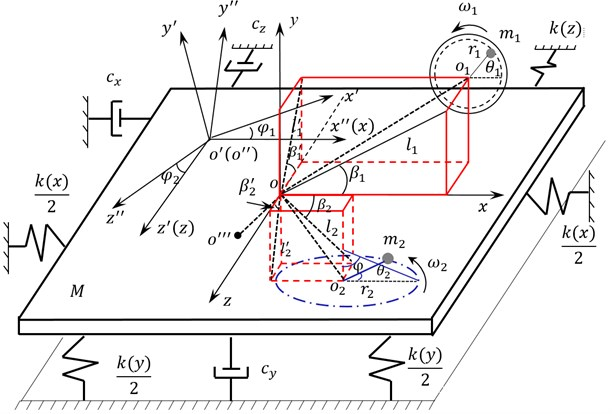### 3.3. Simulation model

It was difficult to get the exact solution of mathematical model from above equations because of the multi variable electromechanical coupling effect. In order to get the vibration characteristics of multi-axis fatigue loading system visually, simulation model based on Matlab/Simulink software was established according to mathematical model. It was showed in Fig. 6. Motor A and Motor B were two loading sources. Every simulation model of AC motor included voltage model, electromagnetic torque model, motion model and flux linkage torque model.

### 3.4. Analysis of simulation test

The simulation model parameters were set as: $M=$25 kg, ${m}_{1}={m}_{2}=$2 kg, ${r}_{1}=$0.15 m, ${r}_{2}=$0.15 m, ${l}_{1}=$0.05 m, ${l}_{2}=$0.25 m, ${l}_{1}^{\mathrm{\text{'}}}=\mathrm{}$0.06 m, ${l}_{2}^{\mathrm{\text{'}}}=\mathrm{}$0.16 m, ${\beta }_{1}={\beta }_{2}=\mathrm{}$14°, ${\beta }_{1}^{\mathrm{\text{'}}}=$ 42°, ${\beta }_{2}^{\mathrm{\text{'}}}=\mathrm{}$48°. The initial phase angle of two loading sources was zero. The initial setting revolution speeds of the loading source were ${n}_{1}=$50 r/min and ${n}_{2}=$55 r/min in flapwise direction. The initial setting revolution speeds of the loading source were ${n}_{3}=$70 r/min and ${n}_{4}=$75 r/min in edgewise direction. ${n}_{1}=$50 r/min and ${n}_{3}=$70 r/min were the same as the nature frequency of blade in flapwise and edgewise direction respectively. The revolution speed and phase angle variable simulation results of the loading source were shown in Fig. 7 and Fig. 8. The amplitude of wind turbine blade and the overall trajectories of the flapwise direction were shown in Fig. 9.

Fig. 6The simulation model of multi-axis excitation system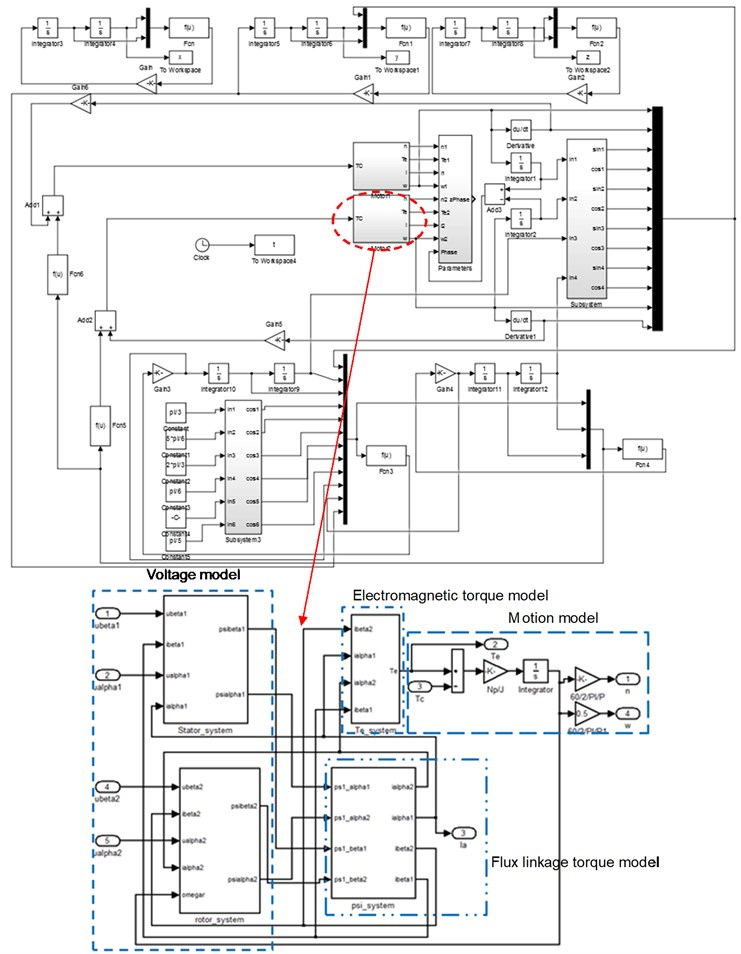Fig. 7Revolution speed and phase angle simulation of loading source in flapwise direction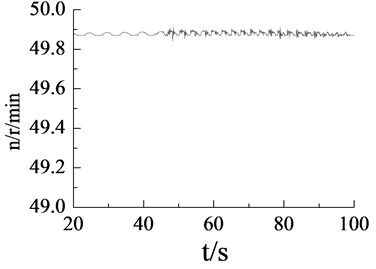a)${n}_{1}=$50 r/min, revolution speed of loading source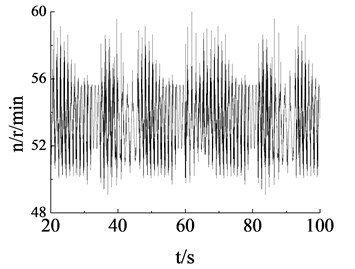b)${n}_{2}=$ 55 r/min, revolution speed of loading source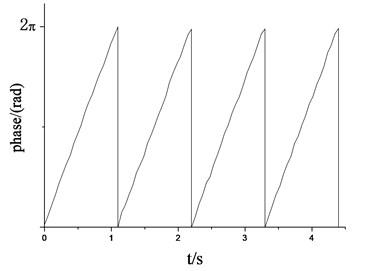c)${n}_{1}=$50 r/min, phase angle of loading source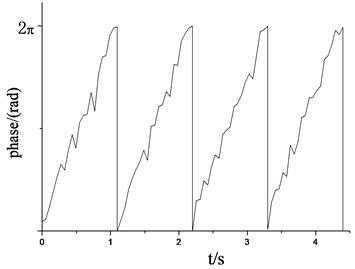d)${n}_{2}=$55 r/min, phase angle of loading source

Fig. 8Revolution speed and phase angle simulation of loading source in edgewise direction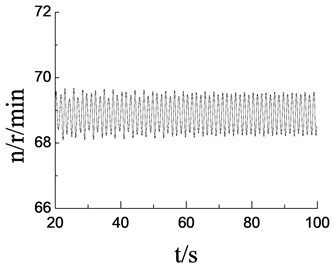a)${n}_{3}=$70 r/min, revolution speed of loading source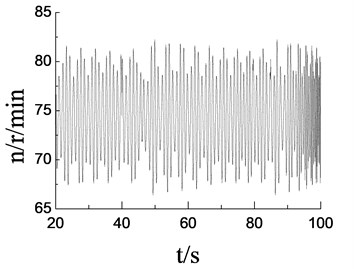b)${n}_{4}=$75 r/min, revolution speed of loading source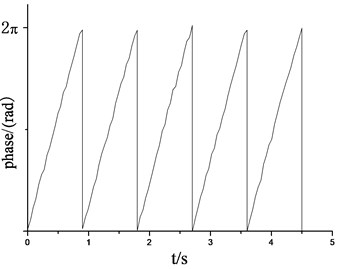c)${n}_{3}=$ 70 r/min, phase angle of loading source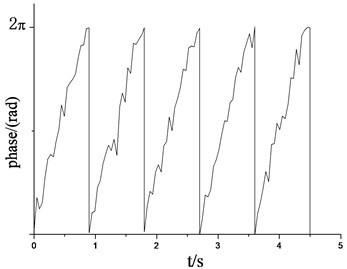d)${n}_{4}=$75 r/min, phase angle of loading source

Fig. 9Vibration trajectory simulation of wind turbine blade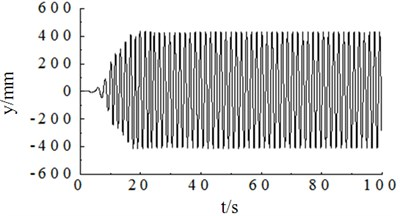a)${n}_{1}=$50 r/min, amplitude simulation curve of flapwise direction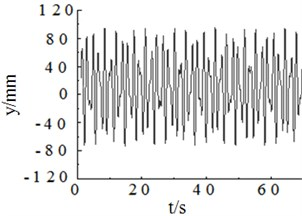b)${n}_{2}=$55 r/min, amplitude simulation curve of flapwise direction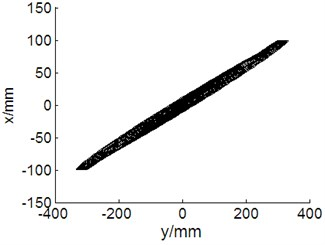c)${n}_{1}=$50 r/min, ${n}_{3}=$70 r/min, vibration trajectory of blade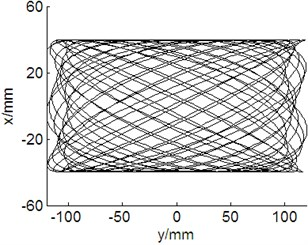d)${n}_{2}=$55 r/min, ${n}_{4}=$ 75 r/min, vibration trajectory of blade

## 4. Test verification

### 4.1. Experiment platform

In order to verify the theoretical analysis and the simulation results, a multi-axis fatigue loading test system of small wind turbine blade was set up. The HMI monitoring interface based on Labview software was developed as shown in Fig. 10.

The whole control system used the distributed network bus mode, which was shown in Fig. 11. Two slave controllers controlling the frequency converter adjusted the revolution speed of every loading source. Laser range devices, measuring the amplitude of the blade through the RS485 bus, transmitted data to the controller. The main controller and the slave controllers exchanged the data through the CAN bus. The main controller and the monitoring interface also used the RS485 bus to realize the data exchange. All useful information was displayed and saved on the monitor interface.

Fig. 10Test platform and interface monitor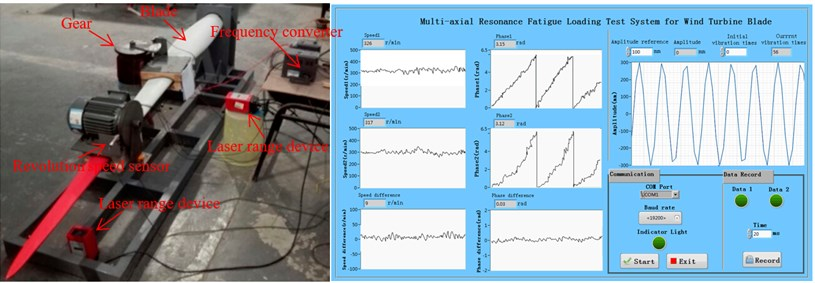Fig. 11Distributed network control architecture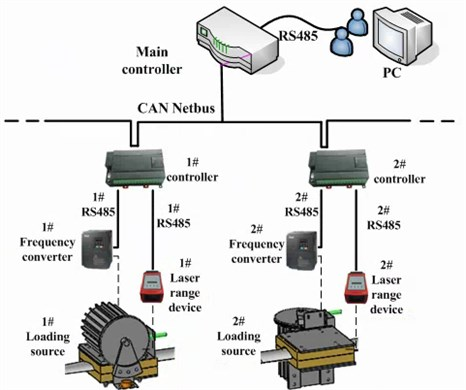Infinon XC164CS MCU was used for control the system hardware. The software in Keil3.0 compiler environment used C language to complete. The measure device for revolution speed was used the high-speed counting sensor and gear. Test parameters were shown in Table 2.

Table 2Test parameters

 Parameters Value Blade length (m) 2.3 Blade weight (kg)1st frequency in flapwise (Hz) 1st frequency in edgewise (Hz) 250.861.15 Eccentric mass (kg) 2 Eccentric length (m) 0.15 Motor power (kW)Frequency converterLaser range deviceSensor type 0.75ABBADSL-30CORON-CTS Test temperature (°C) 23 Humidity(RH) 36 %

### 4.2. Test result and analysis

When the revolution speed of the loading source was respective setting with 50 r/min and 55 r/min in flapwise direction, the revolution speed and phase angle variation of loading source were measured as shown in Fig. 12. According to the test results, when the setting revolution speed was same to the natural frequency of blade ($n=$50 r/min), revolution speed of loading source tends to be stable, and the phase angle varied smoothly. When the setting revolution speed was different with the natural frequency of blade ($n=$55 r/min), due to the coupling effect between them, the revolution speed and phase angle variation of loading source fluctuated significantly. Above test measure results were basically match with the simulation results.

When the revolution speed of the loading source was also respective setting 70 r/min and 75 r/min in edgewise direction, the revolution speed of loading source was measured as shown in Fig. 13. According to the test results, when the setting revolution speed was same with the natural frequency of blade ($n=$70 r/min), revolution speed of loading source tends to be stable and the phase angle varied smoothly. When the setting revolution speed was different with the natural frequency of blade ($n=$75 r/min), due to the coupling effect between them, the revolution speed and phase angle variation of loading source also fluctuated significantly. Above test measure results were also basically match with the simulation results.

Fig. 12Revolution speed and phase angle test curve of loading source in flapwise direction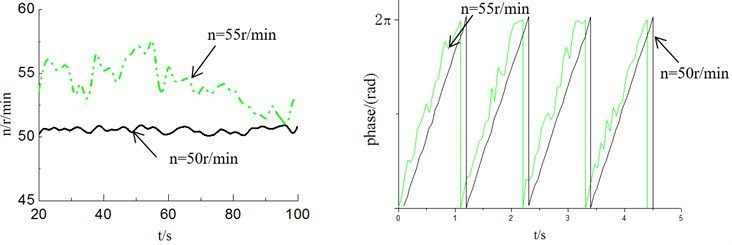Fig. 13Revolution speed and phase angle test curve of loading source in edgewise direction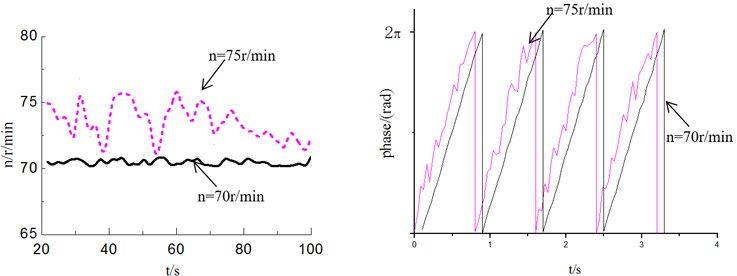Fig. 14Vibration trajectory test curve of wind turbine blade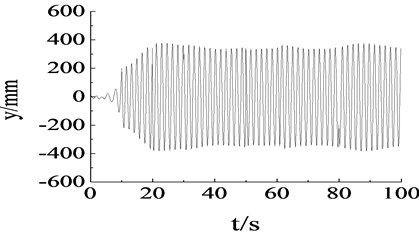a) Amplitude test curve of flapwise direction in resonance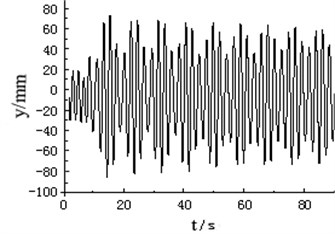b) Amplitude test curve of flapwise direction in non-resonance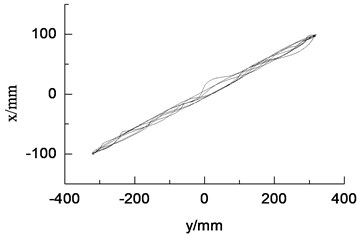c) Vibration trajectory in resonance condition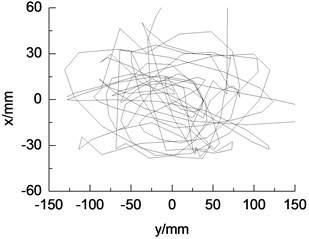d) Vibration trajectory in non-resonance condition

## 5. Conclusions

In this paper, a new multi-axis fatigue loading system of wind turbine blade driven by electric mass was designed. The measurement method for revolution speed, phase angle of loading source and blade amplitude was given. The conclusions were obtained in follows.

1) In order to shorten the measuring time for wind turbine blade fatigue loading test, the multi-axis fatigue loading system based on electric mass driven was designed. By establishing the mathematical model for multi-axis fatigue loading system according to the Lagrange equation, it was found that the proposed system was an electromechanical coupling system. Its numerical solution was very difficult to get.

3) A multi-axis fatigue loading experiment platform was developed. Using this test platform, the test result was basically same with the simulation result, which verified the accuracy of the mathematical model and simulation model in this paper. All the above findings have theoretical and engineering significance for the structure redesign of wind turbine blade

#### Cited by

Aerodynamic characteristic analysis of wind turbine blades based on CSA-KJ airfoil optimization design
Flexible nanocomposite electrothermal films based on carbon nanotubes and waterborne polyurethane with high reliability, stretchability and low-temperature performance for wind turbine blade deicing

27 January 2016
Accepted
04 September 2016
Published
15 February 2017
SUBJECTS
Mechanical vibrations and applications
Keywords